ACT MathHome   Algebra   Geometry   Arithmetic   Trigonometry   Probability/Statistics   Calculus   Mechanics GED   SAT   ACT   College Mathematics   GRE   School Supplies   About Us

ACT Math

 Electronic Math Textbooks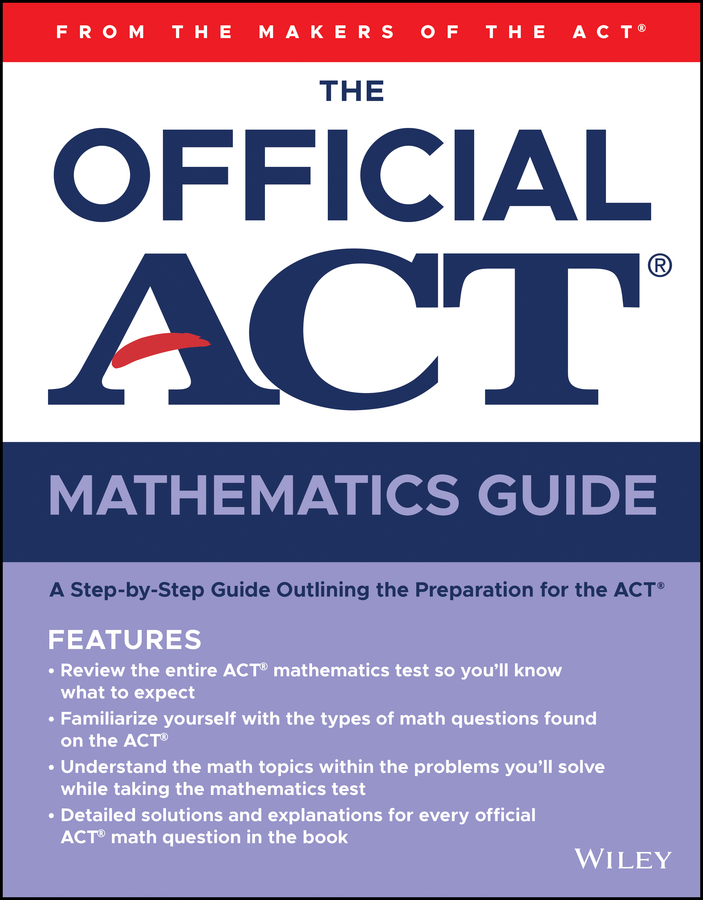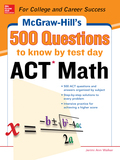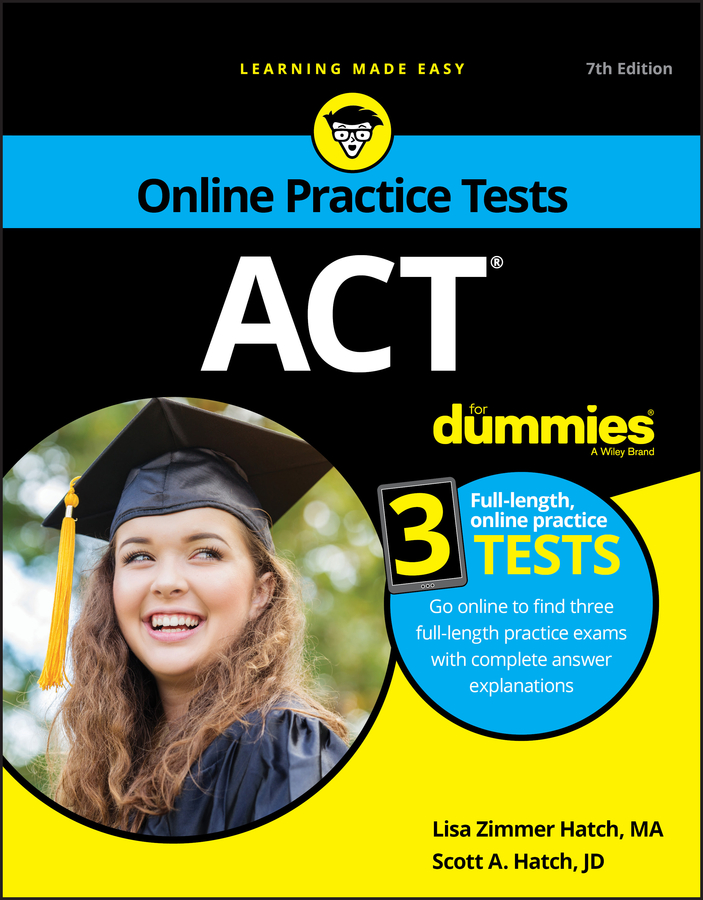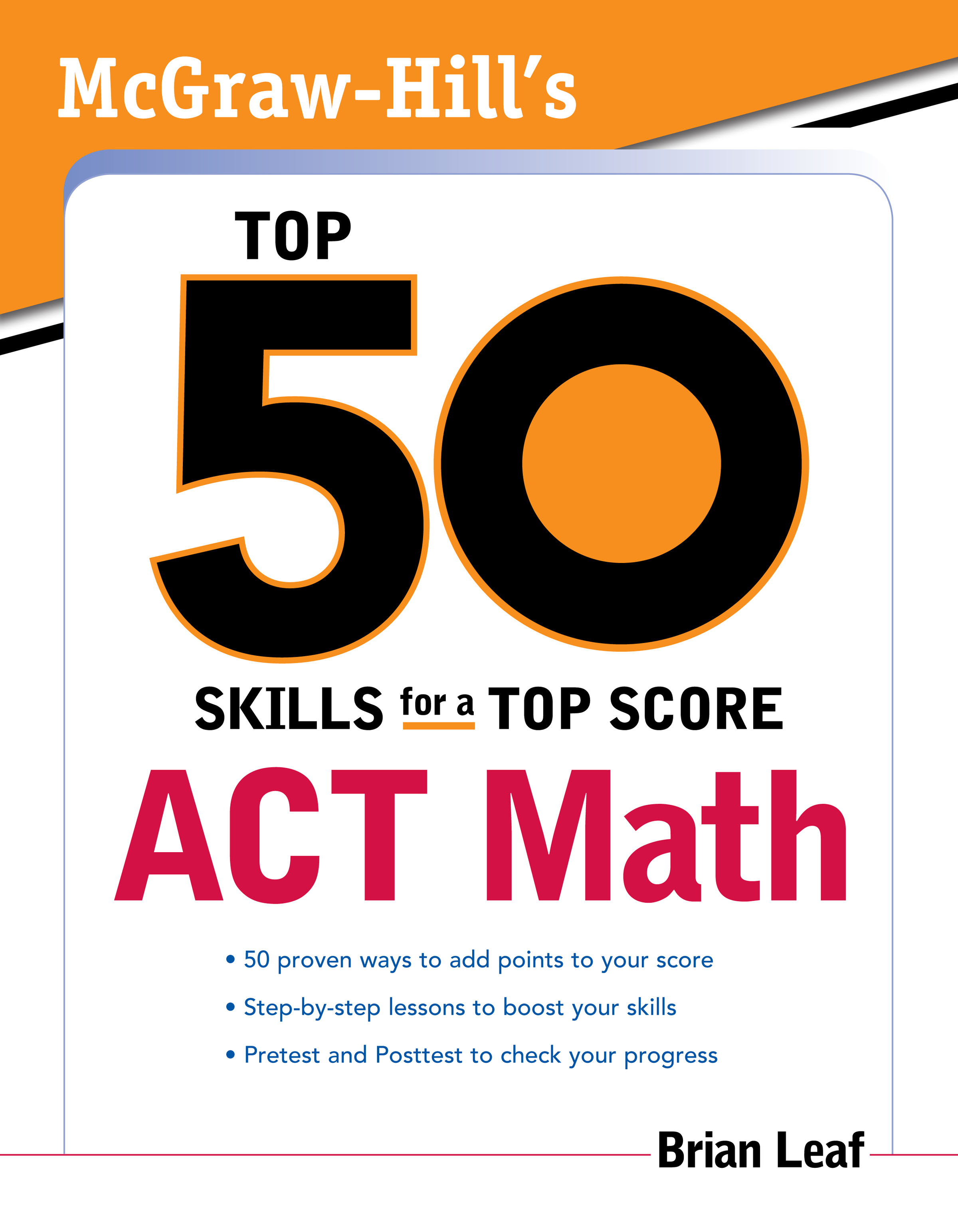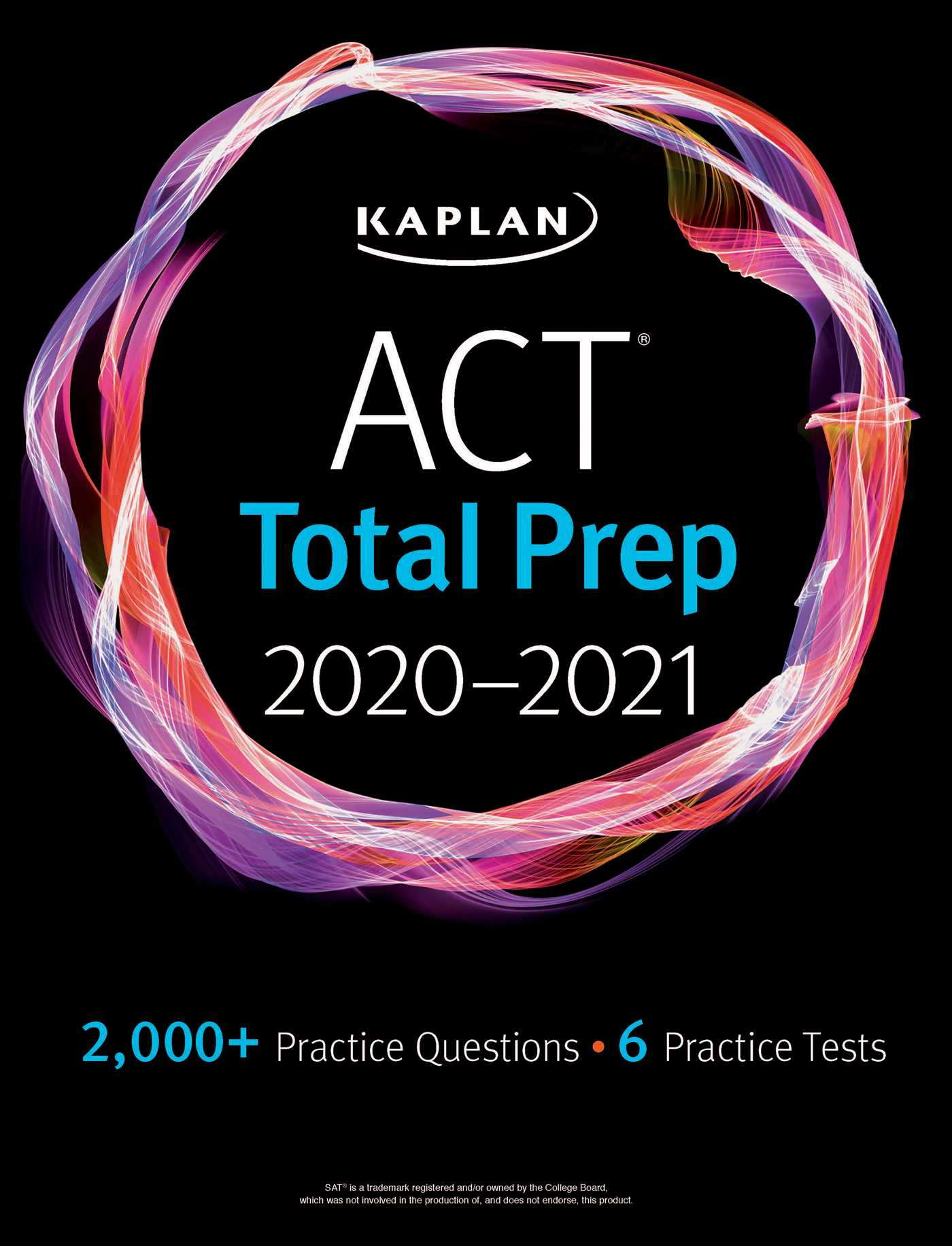Paperback Math Textbooks (New and Used)Overview of the ACT Math Content Pre-AlgebraFactors , Square roots and approximations , Absolute value and ordering numbers by valueExponents, Scientific notation. Elementary Algebra Exponents and square roots, Evaluation of algebraic expressions through substitutionUsing variables to express functional relationships, Algebraic operationsFactoring and quadratic equations Intermediate Algebra Quadratic formula, Rational and radical expressions, Absolute value equations and inequalitiesSequences and patterns, Polynomials, Complex numbers Coordinate Geometry Graphs and their relations with equations including points, lines, polynomials, circles, and other curvesGraphing inequalities, Slope, Parallel and perpendicular lines, Distance; midpoints; and conics Plane GeometryAngles and relations among perpendicular and parallel linesProperties of circles, triangles, rectangles, parallelograms, and trapezoids, TransformationsProof and proof techniques, Volume,  Three-dimensional Geometry Trigonometry Trigonometric relations in right triangles, Values and properties of trigonometric functionsGraphing trigonometric functions, Modeling using trigonometric functionsSolving trigonometric equations Learn more about the

 Trigonometric Equations: Solve the equation, 2sin�θ - sinθ = 0                           This is a Quadratic Equation as in 2x� - x = 0                                          By factorization, Sinθ(2sinθ - 1) = 0                                                 So, sinθ = 0 and 2sinθ - 1 = 0                      When sinθ = 0, angle θ = 0�, 180� and 360�     Also, for 2sinθ - 1 = 0                  sinθ = 0.5. Angle θ = 30� and 150�       Answer: Solutions of θ are 0�, 30�, 150�, 180� and 360�for Math Practice,

 More School Supplies and other useful items *  * *